# Real-Time Implementation of the MPPT Control Algorithms of a Wind Energy Conversion System by the Digital Simulator OPAL_RT

Real-Time Implementation of the MPPT Control Algorithms of a Wind Energy Conversion System by the Digital Simulator OPAL_RT

El Oualid ZouggarSouad Chaouch Lilia Abdelhamid Djaffar Ould Abdeslam

Laboratory of Electromagnetic Induction and Propulsion Systems, University of Batna 2, Rue Chahid Boukhlouf Med El-Hadi 05000, Batna, Algeria

Department of Electrical Engineering of Msila University, BP 166 M’sila 28000, Algeria

IRIMAS Laboratory, Haute Alsace University, 61 Rue Albert Camus F-68093 Mulhouse, France

Corresponding Author Email:
eloualid.zouggar@univ-msila.dz
Page:
45-52
|
DOI:
https://doi.org/10.18280/ejee.230106
1 January 2020
|
Accepted:
20 December 2020
|
Published:
28 February 2021
| Citation

OPEN ACCESS

Abstract:

This paper presents a comparative study between two algorithms for controlling the Wind Turbine (WT) using real time platforms: RT-Lab. The Maximum Power Point Tracking (MPPT) control technique is implemented for extracting the maximum energy from the wind. The first control consists in taking as a reference strategy the electromagnetic torque associated with the maximum power curve. This controller is known as Indirect Speed Control (ISC). The second one, based on the measured wind speed, is called Direct Speed Control (DSC). In this second controller, the effectiveness of the controllers was evaluated with a PI controller and a Fuzzy Logic (FL) controller. The performances are analyzed and compared on the OPAL-RT digital simulator, which is based on the RT-LAB platform with the model, and its control built in Simulink. The results of the simulations clearly show that algorithm based on fuzzy controllers gives better performance in terms of monitoring the maximum power coefficient and optimal speed compared to conventional algorithms.

Keywords:

wind turbine, modeling, MPPT, Real Time Simulation, Opal-RT, Pitch Control, Fuzzy logic

1. Introduction

Today, with the growing demand for electricity and the need to respect the environment, the exploitation of renewable energy sources gives a technical boost to produce sustainable energy that respects the environment. Among these energies, we find wind energy, solar energy etc. The Wind Energy Conversion System (WECS) is one of the renewable energy sources that offers an excellent opportunity to produce electricity with environmental conservation [1, 2]. Converting the kinetic energy of the wind into electrical energy is the role of a wind system. The main components of this system being the turbine, the multiplier, the double-feed induction generator, the converters and its controls [3, 4].

In order to make the WECS operational and to increase its efficiency, a Maximum Power Point Tracking (MPPT) algorithm is required. MPPT is a system used to extract maximum wind power. The main factor influencing the power generated by the WECS is the wind speed. This factor must be taken into consideration during the design of the MPPT algorithm. The main objective of the MPPT is to approximate the mechanical operating speed of the WECS at which the power produced is maximum. MPPT is very necessary in WECS.

In this article, we studied the control of the wind turbine with the application of two MPPT control algorithms. In order to find the reference electromagnetic torque that will be applied by the generator, the first algorithm is based on the measurement of the speed of the generator without servo control of the speed i.e. with an open loop. In the second algorithm, the wind speed was measured to find the reference speed that will be compared with the mechanical speed of the generator, this system is in a closed loop that requires a regulator to obtain the reference electromagnetic torque. First, we apply a PI controller that depends on the system parameters. In the second, and in order to make the controller independent of these parameters, we used a controller based on fuzzy logic. In an effort to circumvent the problem of modeling and system parameters, the Fuzzy Logic Controller (FLC) is preferred, it uses an inference system and human expert knowledge without mathematical models . Adjusting the speed of a WECS by the fuzzy controller requires the choice of the following parameters: linguistic variables, membership functions, inference method and defuzzification strategy [6-8].

To implement these MPPT algorithms, we used Opal-RT Technologies' RT-LAB real-time simulator. RT-LAB generally uses standard PCs with Input and Output (I/O) boards based on FPGA. However, the objective of this paper is to study a comparison between two control algorithms of the wind turbine using real-time platform: RT-Lab. In the next section, we will model the turbine and then apply the two methods of the Maximum Power Point Tracking (MPPT) strategy. Then, after the implementation of the turbine model on the OPAL-RT platform, the real-time simulation results based on the RT_LAB platform will be presented, compared and the performance will be analyzed. Finally, i.e. the last section of, this document will be supplemented by a conclusion.

2. Wind Turbine Model

The power contained in the form of kinetic energy in the wind crossing at a speed Vv and the surface A, is expressed [9, 10] by:

$P_{t}=\frac{1}{2} \rho A V_{v}^{3}$    (1)

where, ρ is the air density (kg/m3).

The wind turbine can recover only a part of that power :

$P_{t}=\frac{1}{2} \rho \pi R_{t}^{2} V_{v}^{3} C_{p}(\lambda, \beta)$    (2)

where, Rt is the radius of the wind turbine and Cp represents the aerodynamic efficiency of the wind turbine. It depends on the blades orientation angle β and the tip speed ratio λ given by [4, 12, 13]:

$\lambda=\frac{\Omega_{t} R_{t}}{V_{v}}$    (3)

where, t is the angular speed of the turbine rotor.

The expression of this power coefficient has been approached for this type of turbine, by the following equation [14-16]:

$C_{p}(\lambda, \beta)=0.73\left(\frac{151}{\lambda_{i}}-0.58 \beta-0.002 \beta^{2.14}-13.2 e^{-\frac{18.4}{\lambda_{i}}}\right)$    (4)

with:

$\frac{1}{\lambda_{i}}=\frac{1}{\lambda+0.02 \beta}-\frac{0.003}{\beta^{3}+1}$    (5)

The coefficient Cp is influenced by two parameters; the speed ratio λ and pitch angle β. Figure 1 shows the relation between Cp, λ and β. It can be seen that the power coefficient Cp can be set to be its maximum value by adjusting both the pitch angle β and the rotation speed of the turbine. The value of λ corresponding to maximum of mechanical power available is called λopt (optimal).

## 1.png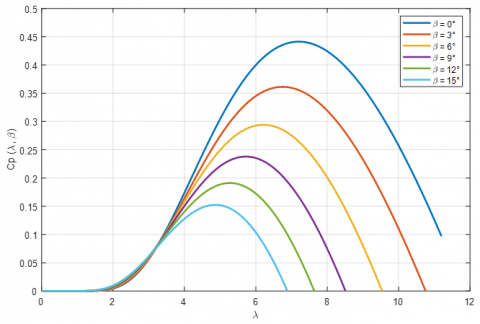Figure 1. Curves of coefficients of power of a 2 MW wind turbine, for different pitch angles β 

The rotor torque is obtained from the power received and the speed of rotation of the turbine [4, 17]:

$T_{t}=\frac{P_{t}}{\Omega_{t}}=\frac{1}{2 \Omega_{t}} \rho \pi R_{t}^{2} V_{v}^{3} C_{p}(\lambda, \beta)$    (6)

The power transmission train is constituted by the blades linked to the hub, coupled to the slow shaft, which is linked to the gearbox, which multiplies the rotational speed of the fast shaft connected to the generator.

The turbine rotational speed and driving torque are expressed in the fast shaft by:

$\Omega_{m e c}=G \Omega_{t}$    (7)

$T_{m e c}=\frac{T_{t}}{G}$    (8)

where, m and t are the generator and turbine speed, Tmec and Tt are the generator and turbine Torque, and G is the gearbox ratio.

Figure 2 shows the variation of the mechanical power available for a type of wind. As can be shown, the maximum power operation point depends also on the wind speed.

## 2.png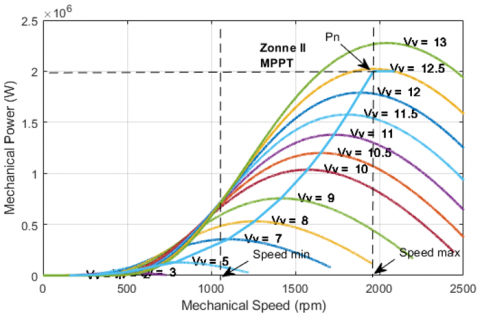Figure 2. Variations of the mechanical power available for a type of wind system

The characteristic corresponding to the maximum power that must be extracted is shown in Figure 3.

## 3.png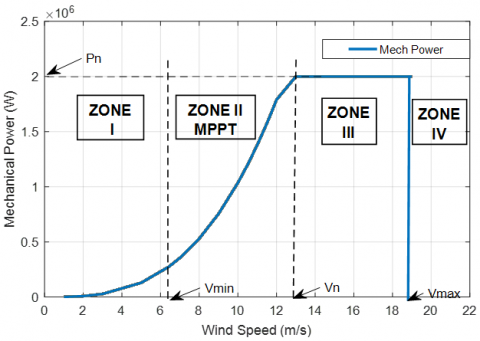Figure 3. Law of optimal ordering of a wind system at variable speed

For every different wind speed there is a different optimal tip speed ratio.

Zone I: corresponds at the very low speeds of the insufficient wind to actuate the wind system.

Zone II: follows the curve of maximum power extraction from variable speed operation with partial load.

Zone III: limits the maximum speed at partial load operation.

Zone IV: corresponds at the very high speeds of the wind for which the number of revolutions of the wind system is limited to a maximum value to avoid damage on the structure.

The dynamic equation of the wind turbine can be given as follows:

$J \frac{d \Omega_{m}}{d t}=T_{m}-T_{e m}-f_{v} \Omega_{m}$    (9)

where, J and fv are the inertia and the friction coefficient of the WECS, and Tem is the generator electromagnetic Torque.

From the previous equations the block diagram corresponds to the aerodynamic and mechanical novelizations of the wind turbine has represented by Figure 4 as follows:

## 4.png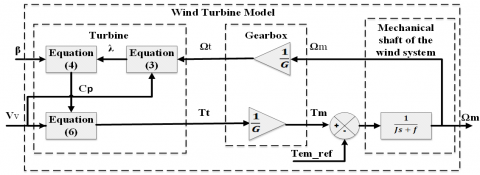Figure 4. Modelling of the mechanical part of the wind turbine

3. Maximum Power Tracking Control

To make better use of the energy available in the wind, wind turbine used for electricity generation must produce maximum power. Therefore, the main objective of the control in Zone 2 is to maximize the capture of wind energy at different wind speeds, which can be achieved by adjusting the rotational speed of the wind turbine by controlling the electromagnetic torque of the generator such that the optimum peak velocity ratio λopt is maintained.

This control is independent of the generator technology and can be simulated without modeling the electrical machine, power converters, and their associated controls just including the torque dynamics as a first order system. Two strategies can be distinguished for controlling maximum power extraction (MPPT); the Indirect Speed Controller without speed control and the Direct Speed Controller with speed control.

3.1 Indirect speed controller

In practice, it is very difficult to measure wind speed because the anemometer is located behind the wind turbine rotor and the diameter swept by the wind turbine blades is very large. For this reason, it uses a non-servo- controlled speed control based on the assumption that wind speed varies very little in steady state in front of the electrical time constants of the wind system, which implies that the acceleration torque of the turbine can be considered as zero.

In addition, if we neglect the effect of torque due to viscous Tvis friction and according to the mechanical Eq. (8) of the wind system, we can write:

$J \frac{d \Omega_{m}}{d t}=T_{m}-T_{e m}-T_{v i s}=0$    (10)

$T_{m}=T_{e m}$    (11)

The principle of building the MPPT control without speed control has based on the measurement of the generator rotation speed, so from this measurement we can estimate the turbine rotation speed as follows:

$\Omega_{t_{-} e s t}=\frac{\Omega_{m e c}}{G}$    (12)

From Eq. (3), the value of the wind speed can be having estimated as follows:

$V_{v_{-} e s t}=\frac{R_{t} \Omega_{t{\text {_est }}}}{\lambda}$    (13)

With the knowledge of an estimate of the turbine rotation speed (12) and the estimate wind speed (13), the torque of the wind turbine is expressed by:

$T_{t_{-} \text {est }}=\frac{1}{2 \Omega_{t_{-} e s t}} \rho A V_{v_{-} e s t}^{3} C_{p}(\lambda, \beta)$    (14)

By replacing Eqns. (12) and (13) in (14), we obtain a relationship of the electromagnetic control torque, such that:

$T_{e m_{-} r e f}=\frac{1}{2 G^{3} \lambda^{3}} \rho \pi R_{t}^{5} C_{p}(\lambda, \beta) \Omega_{m}^{2}$    (15)

In order to obtain the maximum power, it is necessary to set the relative speed to its optimal value of λoptto obtain the maximum power coefficient Cpmax. Therefore, the electromagnetic torque of adjustment is given by:

$T_{e m_{-} r e f}=\frac{1}{2 G^{3} \lambda_{o p t}^{3}} \rho \pi R_{t}^{5} C_{p \max } \Omega_{m}^{2}$    (16)

According to the previous equations, the block diagram of the Figure 5 shows the principle of MPPT control of the wind system without speed control.

## 5.png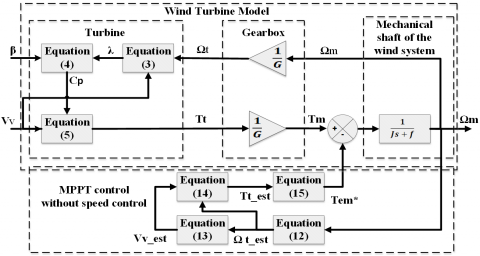Figure 5. MPPT control without speed control

## 6.pngFigure 6. MPPT control with speed control

3.2 Direct control speed

This control method is based on measurements of wind speed and rotation speed of the WECS, and to extract the maximum power from the turbine, the WECS rotation speed must be set to a reference speed. In order to obtain a rotation speed m equal to its reference speed m_ref, the generator must generate an electromagnetic reference torque Tem_ref opposite to the mechanical torque generated by the turbine, which is considered as a disturbing input.

To achieve this torque, the speed control must fulfil two roles in the wind power system:

- The control of the mechanical speed of the rotor to its reference speed.

- Reduction of wind torque action.

In order for the mechanical power to be maximum, the value of the power coefficient Cp (λ, β) must be maximum. This is obtained by setting the value of the velocity relative to its optimal value (λ = λopt).

According to Eq. (3), the reference rotational speed of the turbine has defined as follows:

$\Omega_{t_{-} r e f}=\frac{\lambda_{o p t} V_{v}}{R_{t}}$    (17)

Taking into account the gearbox gain, the reference can be deduced from the rotation speed of the WECS:

$\Omega_{m_{-} r e f}=G \Omega_{t_{-} r e f}$     (18)

The design of this control scheme is illustrated in Figure 6, where the measured wind speed Vv is used to produce the generator speed reference λopt_ref as a function of the optimal peak speed ratio λopt. The generator speed mis controlled by the power converters and will be equal to its reference in established speed, at which the MPPT is reached. In this control strategy and for the control of the rotation speed of the wind system, two algorithms have been applied, which are PI and fuzzy logic.

3.2.1 PID controller

In the industry, the most commonly used process control algorithms are undoubtedly the PI controller, because of its simplicity in terms of structure that can be easily understood and put into practical implementation .

A PI controller calculates an "error" value as the difference between a process variable measured and a desired setpoint .

The controller tries to minimize the error between the setpoint value and the measured value of the speed by adjusting the process control inputs.

The expression of the PI controller is given by:

$T_{\text {em_ref }}=\left(K_{p}+\frac{K_{i}}{s}\right)\left(\Omega_{m_{-} \text {ref }}-\Omega_{m}\right)$    (19)

The gains Kp and Ki are calculated using the pole's compensation.

3.2.2 Fuzzy logic controller

Fuzzy logic is one of the most powerful control methods because its controllers can work with a mathematical model of an imprecise process and can handle nonlinearity . Basically, the use of fuzzy logic for a system is based on three steps which are: fuzzification, fuzzy rule base and defuzzification .

For the MPPT command, the FLC has two inputs and one output. The two input variables of the FLC are the error (E) between the reference speed and the measured speed and the error change (CE) and the output variable is the electromagnetic reference torque (Table 1).

In order to convert the system's input values E and CE into fuzzy language sets fuzzy membership functions are used, this process is called fuzzification. Figure 7 shows the five language labels represent these entries: (such as ZE (zero), PB (positive big), PS (positive small), NB (negative big), and NS (negative small)), and the five language labels represent the output: High Decrease (HD), Low Decrease (LD), Zero (Z), Low Increase (LI), High Increase (HI).

## 7.png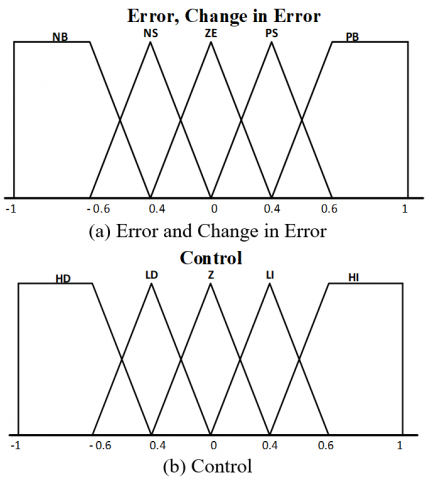Figure 7. Membership for inputs and output

Table 1. Rules table of the fuzzy controller

 E ΔE NB NS ZE PS PB NB HD HD HD LD Z NS HD HD LD Z LI ZE HD LD Z LI HI PM LD Z LI HI HI PB Z LI HI HI HI
4. RT-LAB Platform Simulator and OPAL-RT Digital Simulator

Real-Time Simulation (RTS) has been around for a long time and has been used in many areas of engineering [22, 23]. Therefore, it allows a computer model to be built from a real physical system, which will be run in a computer at the same rate as real time. RT-LAB is an integrated platform for simulation of block diagram models in real time, with fast and automatic implementation. RT-LAB, fully integrated with MATLAB/Simulink®, is the open real-time simulation software environment that has revolutionized the way model-based design is performed. RT-LAB’s flexibility and scalability allow it to be used in virtually any simulation or control system application, and to add computing-power to simulations, where and when it is needed.

After using the Matlab / Simulink for wind turbine modeling and simulation, this model is then used to automatically generate the code, which is then compiled and downloaded to RT-LAB. When the code is generated, it must be uploaded to OPAL-RT for real-time implementation. Figure 8 shows the development diagram of a real-time implementation of the MPPT Control Algorithms of a Wind Energy Conversion System by the digital Simulator OPAL_RT.

## 8.png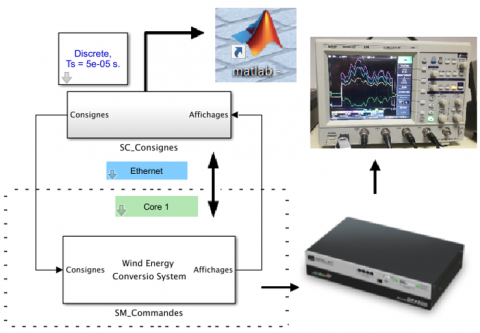Figure 8. Real-time simulation development scheme

5. Results and Discussions

5.1 Matlab simulation

The mathematical model of the wind turbine and its controls was simulated in Matlab-Simulink. Table 2 (See Appendix) presents the parameters of the wind turbine.

Figure 9 represents the variation of the wind speed. We note that this profile was chosen in order to test the behavior of the turbine in two zones: zone II (MPPT Control) and zone III (Pitch Control).

## 9.png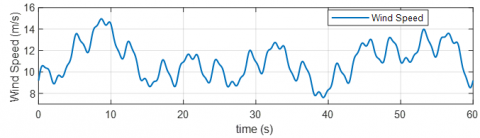Figure 9. Wind speed

## 10a.png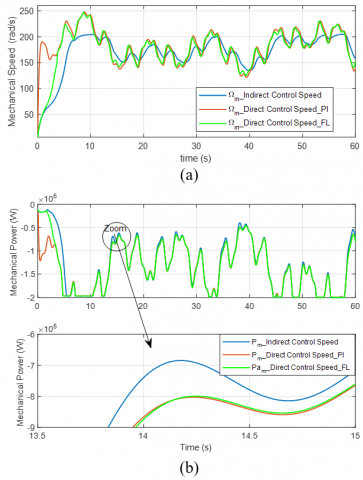## 10b.png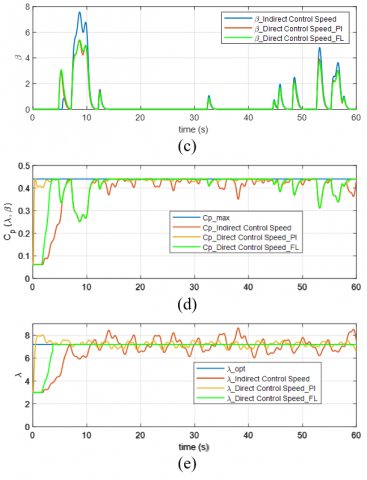Figure 10. Control of wind turbine in zone II and III, (a) mechanical speed, (b) mechanical power, (c) pitch angle variation (β), (d) Power coefficient (Cp) and (e) Tip speed ratio (λ)

Figures 10(a) to 10(e) respectively represent the variation of the mechanical speed, mechanical power, pitch angle β, Cp and λ according to the variation of the wind speed.

We note that during the whole period of zone II, β = 0° the Cp is kept constant at its maximum value which results in an optimal mechanical power for each point of change of wind speed, however, in zone III, β > 0° the mechanical power is maintained at its nominal value (2 MW) and this is due to the control of the pitch angle β resulting from a change in Cp which is reduced.

From the simulation results shown in the figures above, the correct MPPT strategy can be implemented with a fuzzy logic controller. It gives the best response for power coefficient (Cp) and Tip speed ratio (λ), as there is no steady state error, no wobble (snap) and small overshoot (see Figures 10(d) and 10(e)). This controller can be used even if the parameters of wind systems or the wind speed change or are unknown.

Another advantage is the simplicity and low cost of calculating the FL, designed only by fuzzy operations and the necessary rules associated with it.

5.2 Real time simulation

## 11a.png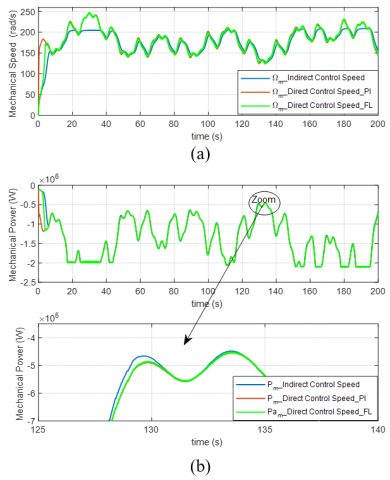## 11b.png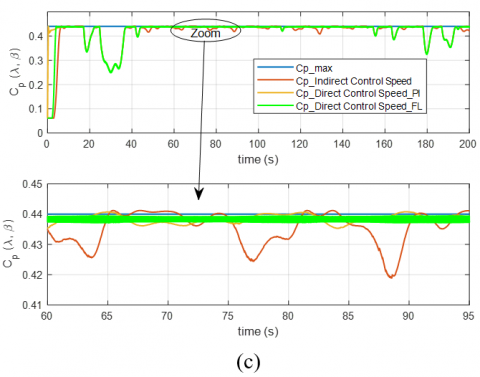## 11c.png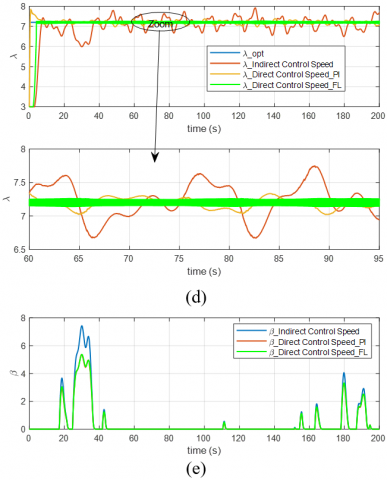Figure 11. Control of wind turbine in zone II and III, (a) mechanical speed, (b) mechanical power, (c) Power coefficient (Cp), (d) Tip speed ratio (λ), (e) pitch angle variation (β)

To execute and test the proposed wind turbine controls in real time, we used the RT-LAB software: V11.2.2.108. Which allows us to send this model to the OPAL-RT platform. After modeling the system in the RT-LAB environment, it was sent to the OP4510 real-time digital simulator with a time step of 50 μs (Figure 11).

6. Conclusion

In this work, we modelled the wind turbine with the use of the WECS mechanical equation. Then, in order to control it, we applied two control strategies to extract the maximum power available in the wind. To validate the proposed commands, we implemented the model studied with its controls in the platform in real time: OPAL-RT.

According to the real-time simulation results obtained, the closed-loop control strategy with the fuzzy controller gives satisfactory dynamic performance compared to the other method.

This work has enabled us to validate the proposed orders in real time and opens the doors for us to implement a complete wind energy conversion system in the future.

Nomenclature
 Vsd,Vsq, Vrd, Vrq Stator and rotor voltage components in the d-q reference frame Isd, Isq ,Ird , Irq Stator and rotor current components in the d-q reference frame Φsd, Φsq, Φrd, Φrq Stator and rotor flux components in the d-q reference frame ωs, ωr Stator frequency, rotor rotating speed p Number of pole pairs Rs, Rr Stator and Rotor resistance respectively Ls, Lr Stator and Rotor inductance respectively Lm Mutual inductance Ps, Qs Active reactive stator power respectively Tem Electromagnetic torque
Appendix

Table 2. Parameters of the wind turbine (2 MW)

 Wind Turbine Parameter Value Unit Nominal Wind Speed 12.5 m/s Maximum power coefficient Cp_max 0.44 - Number of blades 3 - Optimum tip speed ratio λopt 7.2 - Speed multiplier gain G 80 - Rotor Radius Rt 35 m
References

 Domínguez-García, J.L., Gomis-Bellmunt, O., Trilla-Romeroa, L., Junyent-Ferré, A. (2012). Indirect vector control of a squirrel cage induction generator wind turbine. Computers and Mathematics with Applications, 64(2): 102-114. https://doi.org/10.1016/j.camwa.2012.01.021

 Lin, W.M., Hong, C.M., Cheng, F.S. (2010). On-line designed hybrid controller with adaptive observer forvariable-speed wind generation system. Energy, 35(7): 3022-3030. https://doi.org/10.1016/j.energy.2010.03.040

 Pena, R., Clare, J.C., Asher, G.M. (1996). Doubly fed induction generator using back-to-back PWM converters and its application to variablespeed wind-energy generation. IEE Proceedings - Electric Power Applications, 143(3): 231-241. https://doi.org/10.1049/ip-epa:19960288

 Zouggar, E.O., Chaouch, S., Abdeslam, D.O., Abdelhamid, A.L. (2019). Sliding control with fuzzy type-2 controller of wind energy system based on doubly fed induction generator. Instrumentation Mesure Metrologie, 18(2): 137-146. https://doi.org/10.18280/i2m.180207

 Raju, S.K., Pillai, G.N. (2015). Design and implementation of type-2 fuzzy logic controller for DFIG-based wind energy systems in distribution networks. IEEE Transactions on Sustainable Energy, 7(1): 345-353. https://doi.org/10.1109/TSTE.2015.2496170

 Yaakoubi, A.E., Asselman, A., Djebli, A., Aroudam, E.H. (2016). A MPPT strategy based on fuzzy control for a wind energy conversion system. Procedia Technology, 22: 697-704. https://doi.org/10.1016/j.protcy.2016.01.145

 Tiwari, R., Babu, N.R. (2016). Fuzzy logic based MPPT for permanent magnet synchronous generator in wind energy conversion system. IFAC-PapersOnLine, 49(1): 462-467. https://doi.org/10.1016/j.ifacol.2016.03.097

 Nabipour, M., Razaz, M., Seifossadat, S.G., Mortazavi, S.S. (2017). A new MPPT scheme based on a novel fuzzy approach. Renewable and Sustainable Energy Reviews, 74: 1147-1169. https://doi.org/10.1016/j.rser.2017.02.054

 Fdaili, M., Essadki, A., Nasser, T. (2017). Comparative analysis between robust SMC & conventional PI controllers used in WECS based on DFIG. International Journal of Renewable Energy Research (IJRER), 7(4): 2151-2161.

 Islam, M.R., Guo, Y.G., Zhu, J.G. (2010). Steady state characteristic simulation of DFIG for wind power system. In International Conference on Electrical & Computer Engineering (ICECE 2010), Dhaka, Bangladesh, pp. 151-154. https://doi.org/10.1109/ICELCE.2010.5700649

 Nadour, M., Essadki, A., Nasser, T. (2017). Comparative analysis between PI & backstepping control strategies of DFIG driven by wind turbine. International Journal of Renewable Energy Research (IJRER), 7(3): 1307-1316.

 Nunes, M.V.A., Lopes, J.A.P., Zurn, H.H., Bezerra, U.H., Almeida, R.G. (2004). Influence of the variable-speed wind generators in transient stability margin of the conventional generators integrated in electrical grids. IEEE Transactions on Energy Conversion, 19(4): 692-701. https://doi.org/10.1109/TEC.2004.832078

 Rouabhi, R., Abdessemed, R., Aissa, C., Djerioui, A. (2015). Power quality enhancement of grid connected doubly-fed induction generator using sliding mode control. International Review of Electrical Engineering, 10(2): 266-276. https://doi.org/10.13140/RG.2.1.2092.6567

 Jaladi, K.K, Sandhu, K.S. (2019). Real-time simulator based hybrid control of DFIG-WES. ISA Transactions, 93: 325-340. https://doi.org/10.1016/j.isatra.2019.03.024

 Abad, G., Lopez, J., Rodriguez, M., Marroyo, L., Iwanski, G. (2011). Doubly Fed Induction Machine: Modeling and Control for Wind Energy Generation (Vol. 85). John Wiley & Sons.

 Ali, M.M., Youssef, A., Ali, A.S., Abdel-Jaber, G.T. (2019). Comparative study of different pitch angle control strategies for DFIG based on wind energy conversion system. International Journal of Renewable Energy Research (IJRER), 9(1): 157-163.

 Benkahla, M., Taleb, R., Boudjema, Z. (2016). Comparative study of robust control strategies for a dfig-based wind turbine. International Journal of Advanced Computer Science and Applications, 7(2): 455-462. https://doi.org/10.14569/IJACSA.2016.070261

 Mechter, A., Kemih, K., Ghanes, M. (2015). Sliding mode control of a wind turbine with exponential reaching law. Acta Polytechnica Hungarica, 12(3): 167-183. https://doi.org/10.12700/APH.12.3.2015.3.10

 Mudry, F. (2002). Ajustage des paramètres d’un régulateur PID. Ecole d’ingénieurs du Canton de Vaud-Département d’électricité et informatique.

 Kazmierkowski, M.P., Krishnan, R., Blaabjerg, F. (2003). Control in Power Electronics, p. 579. New York: Academic press. https://doi.org/10.1016/B978-0-12-402772-5.X5000-5

 Muyeen, S.M., Al-Durra, A. (2013). Modeling and control strategies of fuzzy logic controlled inverter system for grid interconnected variable speed wind generator. IEEE Systems Journal, 7(4): 817-824. https://doi.org/10.1109/JSYST.2013.2239893

 Wikipedia. (2013). Real-time simulation. Available at http://en.wikipedia.org/wiki/Realtime_simulation, accessed on 21 February 2014.

 Bélanger, J., Venne, P., Paquin, J.N. (2010). The what, where and why of real-time simulation. Planet Rt, 1(1): 25-29.